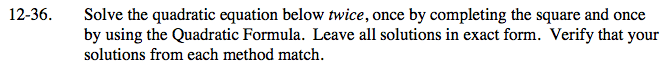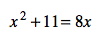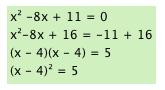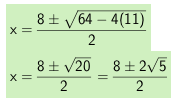### Home > CAAC > Chapter 12 > Lesson 12.2.1 > Problem12-36

12-36.

Solve the quadratic equation below twice, once by completing the square and once by using the Quadratic Formula. Leave all solutions in exact form. Verify that your solutions from each method match. Homework Help ✎x2 + 11 = 8xTake the square root of both sides.

Move all of the terms to one side of the equation
and substitute the numbers into the Quadratic Formula.

a = 1 b = -8 c = 11